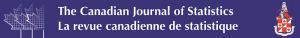# Layman’s abstract for paper on variable ordination of Cholesky‐based estimation for a sparse covariance matrix

Each week, we will be publishing layman’s abstracts of new articles from our prestigious portfolio of journals in statistics. The aim is to highlight the latest research to a broader audience in an accessible format.

The article featured today is from the Canadian Journal of Statistics, with the full article now available to read in Early View here.Kang, X. and Deng, X. (2020), On variable ordination of Cholesky‐based estimation for a sparse covariance matrix. Can J Statistics. doi:10.1002/cjs.11564

In the modern data analytics, data with large number of variables often occur in various scientific areas, such as genetics, finance, social network, fMRI analysis. The covariance matrix, which characterizes the pairwise covariance among variables, plays an important role for modeling and inference. However, the estimation of covariance matrix in the high-dimensional data encounters two challenges. One is that the number of unknown parameters in the matrix increases quadratically in terms of the matrix dimensionality. The other one is the positive definiteness constraint, which is a basic property of a covariance matrix. The modified Cholesky decomposition (MCD) is an efficient technique for large covariance matrix estimation to overcome the above two challenges, but often depends on the order of variables. It implies that different variable orders would lead to different Cholesky-based estimates of covariance matrix. In many cases, the variable order is not available or cannot be pre-determined in practice before data analysis. In this work, we thus address the order issue by considering a set of covariance matrix estimates obtained from different orders of variables used in the MCD. Then we consider an ensemble estimator as the “center” of such covariance matrix estimates in the sense of the Frobenius norm. The sparse structure in the proposed estimator is achieved by imposing a Lasso-type penalty on the objective. The alternating direction method of multipliers algorithm is developed to solve the estimator, which is an iterative procedure until convergence. The proposed method is able to capture the underlying sparse structure of the covariance matrix, and ensures the estimator to be positive definite. Simulation and an analysis of real cancer data are conducted to illustrate the merits of the proposed method.